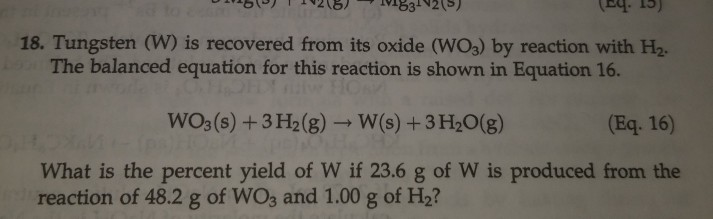# 15 218219831215) (04. 15) 18. Tungsten (W) is recovered from its oxide (WO3) by reaction with H2. The balanced equat...

###### Question:15 218219831215) (04. 15) 18. Tungsten (W) is recovered from its oxide (WO3) by reaction with H2. The balanced equation for this reaction is shown in Equation 16. WO3(s) + 3H2(g) - W(s) + 3 H2O(g) (Eq. 16) What is the percent yield of W if 23.6 g of W is produced from the reaction of 48.2 g of WO3 and 1.00 g of H2?